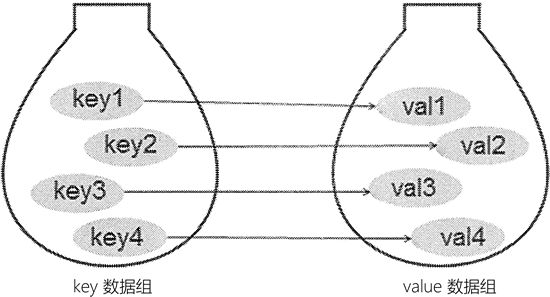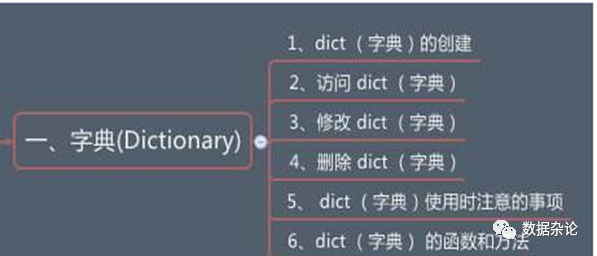# 遍历key和value_python中字典dict的操作技巧汇总

XAMPP案例 1306浏览

``````>>> a = {'one':1, 'tow':2, 'three':3}
>>> a
{'one': 1, 'tow': 2, 'three': 3}
>>> type(a)
<class 'dict'>``````

key和value之间用冒号分隔，多个键值对用逗号分隔。上述字典用`dict`函数创建的代码如下

``````>>> a = dict((('one',1), ('tow',2), ('three',3)))
>>> a
{'one': 1, 'tow': 2, 'three': 3}
>>>
>>> a = dict(zip(['one', 'tow', 'three'], [1, 2, 3]))
>>> a
{'one': 1, 'tow': 2, 'three': 3}
>>>
>>>
>>> a = dict({'one':1, 'tow':2, 'three':3})
>>> a
{'one': 1, 'tow': 2, 'three': 3}``````

``````>>> a = {'one':1, 'tow':2, 'three':3}
>>> a['one']
1``````

#### 1. 访问字典元素

``````# 方括号加下标
>>> a = {'one':1, 'tow':2, 'three':3}
>>> a['one']
1
# get方法
>>> a.get('one')
1``````

#### 2. 修改字典元素

``````# 可以重新赋值，也可以新增值
>>> a = {'one':1, 'tow':2, 'three':3}
>>> a['one'] = 2
>>> a
{'one': 2, 'tow': 2, 'three': 3}
# del 删除元素
>>> del a['one']
# pop删除元素
>>> a.pop("tow")
# 清空字典
>>> a.clear()``````

#### 3. 遍历字典

``````# 返回所有key的列表
>>> a.keys()
dict_keys(['one', 'tow', 'three'])
# 返回所有values的列表
>>> a.values()
dict_values([1, 2, 3])
# 返回(key, value)构成的元组序列
>>> a.items()
dict_items([('one', 1), ('tow', 2), ('three', 3)])
# 返回所有key的列表
>>> list(a)
['one', 'tow', 'three']
# 返回所有key构成的迭代器
>>> iter(a)
<dict_keyiterator object at 0x035A9D20>
>>> for i in iter(a):
...     print(i)
...
one
tow
three``````

``````>>> a = {(1, 2):'one', (3, 4):'tow'}
>>> a[(1,2)]
'one'``````

#### 1. 值为列表的字典

python中不仅key很灵活，其value也很灵活。比如我们想要创建一个value为列表的字典，也是可以的

``````>>> a = {'one' : [1, 2, 3, 4, 5], 'tow':[1, 2, 3, 4, 5]}
>>> a
{'one': [1, 2, 3, 4, 5], 'tow': [1, 2, 3, 4, 5]}
>>> a['one']
[1, 2, 3, 4, 5]``````

``````>>> num = [1, 2, 3, 4, 5]
>>> a = {}
# 直接添加，报错
>>> for i in num:
...     a['one'].append(i)
...
Traceback (most recent call last):
File "<stdin>", line 2, in <module>
KeyError: 'one'
# 先设定为空列表，在追加
# 结果ok
>>> a['one'] = []
>>> for i in num:
...     a['one'].append(i)
...
>>>
>>> a
{'one': [1, 2, 3, 4, 5]}``````

``````>>> from  collections import defaultdict
>>> # 这里的list表明key的默认value为list
>>> a = defaultdict(list)
>>> for i in num:
...     a['one'].append(i)
...
>>>
>>> a
defaultdict(<class 'list'>, {'one': [1, 2, 3, 4, 5]})``````

#### 2. 嵌套的字典

a[key1][key2] = value

``````>>> a = defaultdict(dict)
>>> a['one']['tow'] = 1
>>> a['one']['three'] = 2
>>> a
defaultdict(<class 'dict'>, {'one': {'tow': 1, 'three': 2}})```````weight0 = dict()` print(weight0) # {}

 `weight={'小明':79, '小红':45, '张三':60}` print(weight) {‘小明’: 79, ‘小红’: 45, ‘张三’: 60}

需要指出的是，元组可以作为 dict 的 key，但列表不能作为元组的 key。这是由于 dict 要求 key 必须是不可变类型，但列表是可变类型，因此列表不能作为元组的 key。访问也是通过元组进行访问。

 `# 使用元组作为dict的keydict2 = {(20, 30):'good', 30:'bad'}` print(dict2) {(20, 30): ‘good’, 30: ‘bad’} dict2[(20, 30)] ‘good’

在使用 dict() 函数创建字典时，可以传入多个列表或元组参数作为 key-value 对，每个列表或元组将被当成一个 key-value 对，因此这些列表或元组都只能包含两个元素。例如如下代码：

 `dict_values=[('小明',79),('小红',45),('张三',60)]weight=dict(dict_values)` print(weight) {‘小明’: 79, ‘小红’: 45, ‘张三’: 60}

 `dict0 = dict(a = 10, b = 20.9)` print(dict0） {‘a’: 10, ‘b’: 20.9}

 `weight['小明']7`

 `weight['李四']=90` print(weight） {‘小明’: 79, ‘小红’: 45, ‘张三’: 60, ‘李四’: 90}

 `weight['王五']=130print(weight)del weight['王五']` print(weight) {‘小明’: 79, ‘小红’: 45, ‘张三’: 60, ‘李四’: 60, ‘王五’: 130} {‘小明’: 79, ‘小红’: 45, ‘张三’: 60, ‘李四’: 60}

 `weight['李四']=60` print(weight） {‘小明’: 79, ‘小红’: 45, ‘张三’: 60, ‘李四’: 60}

key的判断

 `print('王五' in weight)` print(‘李四‘ in weight) False True

clear()

clear() 用于清空字典中所有的 key-value 对，对一个字典执行 clear() 方法之后，该字典就会变成一个空字典。

 `dict0 = dict(a = 10, b = 20.9)print(dict0)dict0.clear()` print(dict0) {‘a’: 10, ‘b’: 20.9} {}

get()方法

get() 方法其实就是根据 key 来获取 value，它相当于方括号语法的增强版，当使用方括号语法访问并不存在的 key 时，字典会引发 KeyError 错误；

 `print(weight.get('张三'))` print(weight.get(‘阿大‘)) 60 None

update()方法

update() 方法可使用一个字典所包含的 key-value 对来更新己有的字典。在执行 update() 方法时，如果被更新的字典中己包含对应的 key-value 对，那么原 value 会被覆盖；如果被更新的字典中不包含对应的 key-value 对，则该 key-value 对被添加进去。

 `print(weight)weight.update({'张三':56,'阿小':40})` print(weight) {‘小明’: 79, ‘小红’: 45, ‘张三’: 60, ‘李四’: 60} {‘小明’: 79, ‘小红’: 45, ‘张三’: 56, ‘李四’: 60, ‘阿小’: 40}

items()、keys()、values()

items()、keys()、values() 分别用于获取字典中的所有 key-value 对、所有 key、所有 value。

 `print(weight.items())print(weight.keys())` print(weight.values()) dict_items([(‘小明’, 79), (‘小红’, 45), (‘张三’, 56), (‘李四’, 60), (‘阿小’, 40)]) dict_keys([‘小明’, ‘小红’, ‘张三’, ‘李四’, ‘阿小’]) dict_values([79, 45, 56, 60, 40])

 `for key,value in weight.items():` print(‘Key:{},value:{}’.format(key,value)) Key:小明,value:79 Key:小红,value:45 Key:张三,value:56 Key:李四,value:60 Key:阿小,value:40

 `for (key,value) in zip(weight.keys(),weight.values()):` print(‘Key:{},value:{}’.format(key, value)) Key:小明,value:79 Key:小红,value:45 Key:张三,value:56 Key:李四,value:60 Key:阿小,value:40

 `score = { '小明':{ '语文':99, '数学':99, '英语':98, }, '小红': { '语文': 91, '数学': 89, '英语': 78, }, '小张': { '语文': 59, '数学': 90, '英语': 91, }}score['小明']['数学'] = '100'` print(score) {‘小明’: {‘语文’: 99, ‘数学’: ‘100’, ‘英语’: 98}, ‘小红’: {‘语文’: 91, ‘数学’: 89, ‘英语’: 78}, ‘小张’: {‘语文’: 59, ‘数学’: 90, ‘英语’: 91}}

比如有份体重表，小明：79kg，小红：45kg，张三：60kg，这组数据看上去像两个列表，但这两个列表的元素之间有一定的关联关系。如果单纯使用两个列表来保存这组数据，则无法记录两组数据之间的关联关系。

为了保存具有映射关系的数据，Python 提供了字典，字典相当于保存了两组数据，其中一组数据是关键数据，被称为 key；另一组数据可通过 key 来访问，被称为 value。形象地看，字典中 key 和 value 的关联关系如图 1 所示：图 1 字典保存的关联数据

由于字典中的 key 是非常关键的数据，而且程序需要通过 key 来访问 value，因此字典中的 key 不允许重复。
程序既可使用花括号语法来创建字典，也可使用 dict() 函数来创建字典。实际上，dict 是一种类型，它就是 Python 中的字典类型。
在使用花括号语法创建字典时，花括号中应包含多个 key-value 对，key 与 value 之间用英文冒号隔开；多个 key-value 对之间用英文逗号隔开。`weight0 = dict()` print(weight0) # {}

 `weight={'小明':79, '小红':45, '张三':60}` print(weight) {‘小明’: 79, ‘小红’: 45, ‘张三’: 60}

需要指出的是，元组可以作为 dict 的 key，但列表不能作为元组的 key。这是由于 dict 要求 key 必须是不可变类型，但列表是可变类型，因此列表不能作为元组的 key。访问也是通过元组进行访问。

 `# 使用元组作为dict的keydict2 = {(20, 30):'good', 30:'bad'}` print(dict2) {(20, 30): ‘good’, 30: ‘bad’} dict2[(20, 30)] ‘good’

在使用 dict() 函数创建字典时，可以传入多个列表或元组参数作为 key-value 对，每个列表或元组将被当成一个 key-value 对，因此这些列表或元组都只能包含两个元素。例如如下代码：

 `dict_values=[('小明',79),('小红',45),('张三',60)]weight=dict(dict_values)` print(weight) {‘小明’: 79, ‘小红’: 45, ‘张三’: 60}

 `dict0 = dict(a = 10, b = 20.9)` print(dict0） {‘a’: 10, ‘b’: 20.9}

 `weight['小明']7`

 `weight['李四']=90` print(weight） {‘小明’: 79, ‘小红’: 45, ‘张三’: 60, ‘李四’: 90}

 `weight['王五']=130print(weight)del weight['王五']` print(weight) {‘小明’: 79, ‘小红’: 45, ‘张三’: 60, ‘李四’: 60, ‘王五’: 130} {‘小明’: 79, ‘小红’: 45, ‘张三’: 60, ‘李四’: 60}

 `weight['李四']=60` print(weight） {‘小明’: 79, ‘小红’: 45, ‘张三’: 60, ‘李四’: 60}

key的判断

 `print('王五' in weight)` print(‘李四‘ in weight) False True

clear()

clear() 用于清空字典中所有的 key-value 对，对一个字典执行 clear() 方法之后，该字典就会变成一个空字典。

 `dict0 = dict(a = 10, b = 20.9)print(dict0)dict0.clear()` print(dict0) {‘a’: 10, ‘b’: 20.9} {}

get()方法

get() 方法其实就是根据 key 来获取 value，它相当于方括号语法的增强版，当使用方括号语法访问并不存在的 key 时，字典会引发 KeyError 错误；

 `print(weight.get('张三'))` print(weight.get(‘阿大‘)) 60 None

update()方法

update() 方法可使用一个字典所包含的 key-value 对来更新己有的字典。在执行 update() 方法时，如果被更新的字典中己包含对应的 key-value 对，那么原 value 会被覆盖；如果被更新的字典中不包含对应的 key-value 对，则该 key-value 对被添加进去。

 `print(weight)weight.update({'张三':56,'阿小':40})` print(weight) {‘小明’: 79, ‘小红’: 45, ‘张三’: 60, ‘李四’: 60} {‘小明’: 79, ‘小红’: 45, ‘张三’: 56, ‘李四’: 60, ‘阿小’: 40}

items()、keys()、values()

items()、keys()、values() 分别用于获取字典中的所有 key-value 对、所有 key、所有 value。

 `print(weight.items())print(weight.keys())` print(weight.values()) dict_items([(‘小明’, 79), (‘小红’, 45), (‘张三’, 56), (‘李四’, 60), (‘阿小’, 40)]) dict_keys([‘小明’, ‘小红’, ‘张三’, ‘李四’, ‘阿小’]) dict_values([79, 45, 56, 60, 40])

 `for key,value in weight.items():` print(‘Key:{},value:{}’.format(key,value)) Key:小明,value:79 Key:小红,value:45 Key:张三,value:56 Key:李四,value:60 Key:阿小,value:40

 `for (key,value) in zip(weight.keys(),weight.values()):` print(‘Key:{},value:{}’.format(key, value)) Key:小明,value:79 Key:小红,value:45 Key:张三,value:56 Key:李四,value:60 Key:阿小,value:40

 `score = { '小明':{ '语文':99, '数学':99, '英语':98, }, '小红': { '语文': 91, '数学': 89, '英语': 78, }, '小张': { '语文': 59, '数学': 90, '英语': 91, }}score['小明']['数学'] = '100'` print(score) {‘小明’: {‘语文’: 99, ‘数学’: ‘100’, ‘英语’: 98}, ‘小红’: {‘语文’: 91, ‘数学’: 89, ‘英语’: 78}, ‘小张’: {‘语文’: 59, ‘数学’: 90, ‘英语’: 91}}